# 云栖社区专家系列课——Java必修课第四讲

+关注继续查看

Java必须课是零基础Java学习者的入门课程，涵盖了Java初学者应该掌握的所有核心知识。在第四讲中，最课程创始人、微软MVP陆敏枝详细讲解了基础类型转换、char类型运算、关系运算符、逻辑运算符、位运算符等基础类型之间的运算关系，并结合利息计算器应用案例将前几讲学习的知识点进行串联。

Java必修课在线直播视频链接：

·自动转换：从低精度->高精度byteshortintlongfloatdouble

·强制转换：从高精度->低精度，则要类型强制转换。

Public class OperatorTester{
public static void main(String[] args){
int a = 3;
float c = 5.17f;
c=a;//自动转换
System.out.println(c);//
a=(int)c;//强制转换
System.out.println(a);
}
} 

Javachar类型的运算要注意以下三点：

·char与整型进行算术运算后，结果会自动提升成int类型；

·两个char型运算时，自动转换为int型；

·当char与别的类型运算时，也会先自动转换为int型的，再做其它类型的自动转换。

Public class OperatorTester01{
public static void main(String[] args){
char c1 = 'a'; // 对应97
int f = c1 + 10;
System.out.println("char与整型进行运算: 'a' + 10 = " + f);
//char类型之间运算
char c2 = 'b';  // 对应98
System.out.println("两个char相加: 'a' + 'b' = " + (c1 + c2));
//char与其他类型之间的运算
double db = c1 + 1.1d;//双精度double
System.out.println("char与别的类型相加 : 'a' + 1.1d = " + db);}
}

char与 整型进行运算: 'a' + 10 = 107

char与别的类型相加 : 'b' + 1.1d = 98.1

ASC码表中规定了char类型与整数值的对应关系，具体关系如图：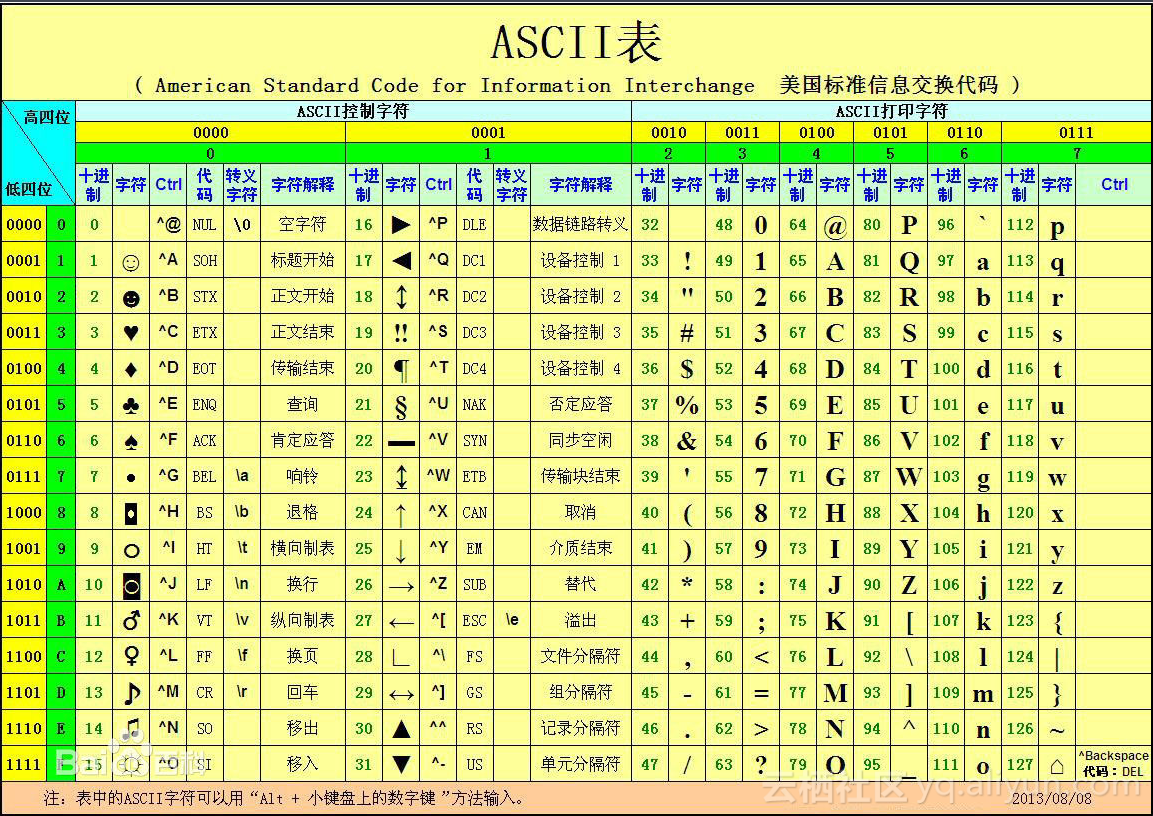public static void m3() {
int a = 1;
int b = 3;
int c = 3;
float d = 3.0f;
char c1 = 'a';
char c2 = 'b';
System.out.println("1 == 3 吗，" + ( a == b));
System.out.println("3 == 3 吗，" + ( b == c));
System.out.println("3 == 3.0 吗，" + ( c == d));
System.out.println("1 != 3 吗，" + ( a != b));
System.out.println("3 > 1 吗，" + ( b > a));
System.out.println("3 >= 3 吗，" + ( b >= a));
System.out.println("3 < 1 吗，" + ( b < a));
System.out.println("3 <= 1 吗，" + ( b <= a));
System.out.println("'a' <= 'b' 吗，" + ( c1 < c2));
}

1 == 3 吗，false

3 == 3 吗，true

3 == 3.0 吗，true

1 != 3 吗，true

3 > 1 吗，true

3 >= 3 吗，true

3 < 1 吗，false

3 <= 1 吗，false

'a' <='b' 吗，true

1== 号和 = 的区别；

2char类型同样可以比较大小。

Public class OperatorTester03{
public static void main(String[] args){
boolran a= true;
boolran a=false;
System.out.println("a && b"+(a&&b));
System.out.println("a || b"+(a||b));
System.out.println("!a" +(!a));
System.out.println("a ^ b"+(a^b));
System.out.println("a & b"+(a&b));
System.out.println("a | b"+(a|b));
}
}

a&&b=false

a||b=true

!a=false

a^b=true

a&b=true

a|b=true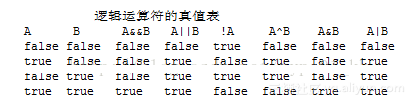AB是逻辑运算的两个逻辑变量，从真值表中可以看出：

1A&&B A&B 结果完全相同，而 A||BA|B结果也完全相同；

2对于&&运算来说，只要运算符左端的值为false，则因无论运算符右端的值为true或为false，其最终结果都为false所以，系统一旦判断出&&运算符左端的值为false，则系统将终止其后的计算过程；因此，&&的效率就比&高，因为&进行了一些无用的并且还不影响结果的计算。

3对于 || 运算来说，只要运算符左端的值为true，则因无论运算符右端的值为true或为false，其最终结果都为true。所以，系统一旦判断出|| 运算符左端的值为true，则系统将终止其后的计算过程。因此，||的效率就比|高，因为&进行了一些无用的并且还不影响结果的计算。

A||BA&&B这种提前结束的行为叫做：短路。

1、十进制计算方法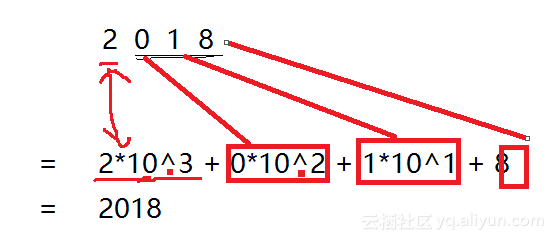2、二进制计算方法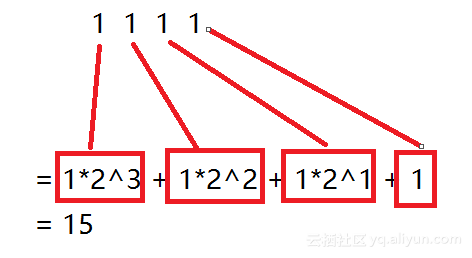15 & 6

Public class OperatorTester04{
public static void main(String[] args){
int x = 15;
int y = 6;
int z = 0;
//x等于二进制数的00001111
//y等于二进制数的00000110
//&等于二进制数的00000110= 2^2 + 2^1=6
z = x & y;
System.out.println("x & y = " + z);
}
}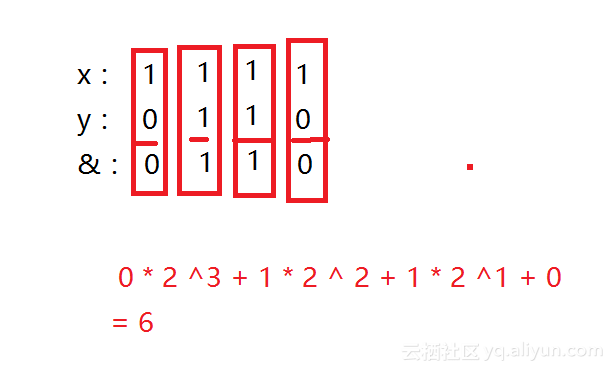3、位运算

·&运算：对二进制的位求与，参考真值表

·| 运算：对二进制的位求或，参考真值表

·~运算：求非

·^运算：求位异或。

·>>运算：将一个数化成二进制右移

·<<运算：将一个数化成二进制左移

·>>>运算：右移（补零）运算符，即无符号右移，">>>"永远不会产生负号，因为其符号位总是被补零。

1赋值运算符=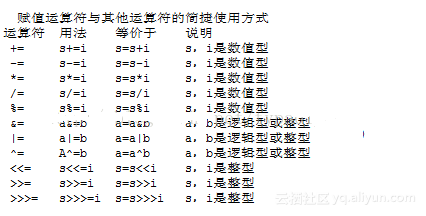2方括号[]

3、三元运算符

Public class OperatorTester05{
public static void main(String[] args){
int c;
c= 1 > 2 ? 1 : 2; //?前表达式为true，取值为:前面值，否则为后面的值
System.out.println(c);
}
}

4、强制类型转换符()

Public class OperatorTester06{
public static void main(String[] args){
int a;
double b = 5.66600;
a = (int) b;
System.out.println(a);

5、对象运算符instanceof

Public class OperatorTester07{
public static void main(String[] args){
String s = new String("str");
if(s instanceof Object){
System.out.println("s is object ");
}
}
}

7点运算符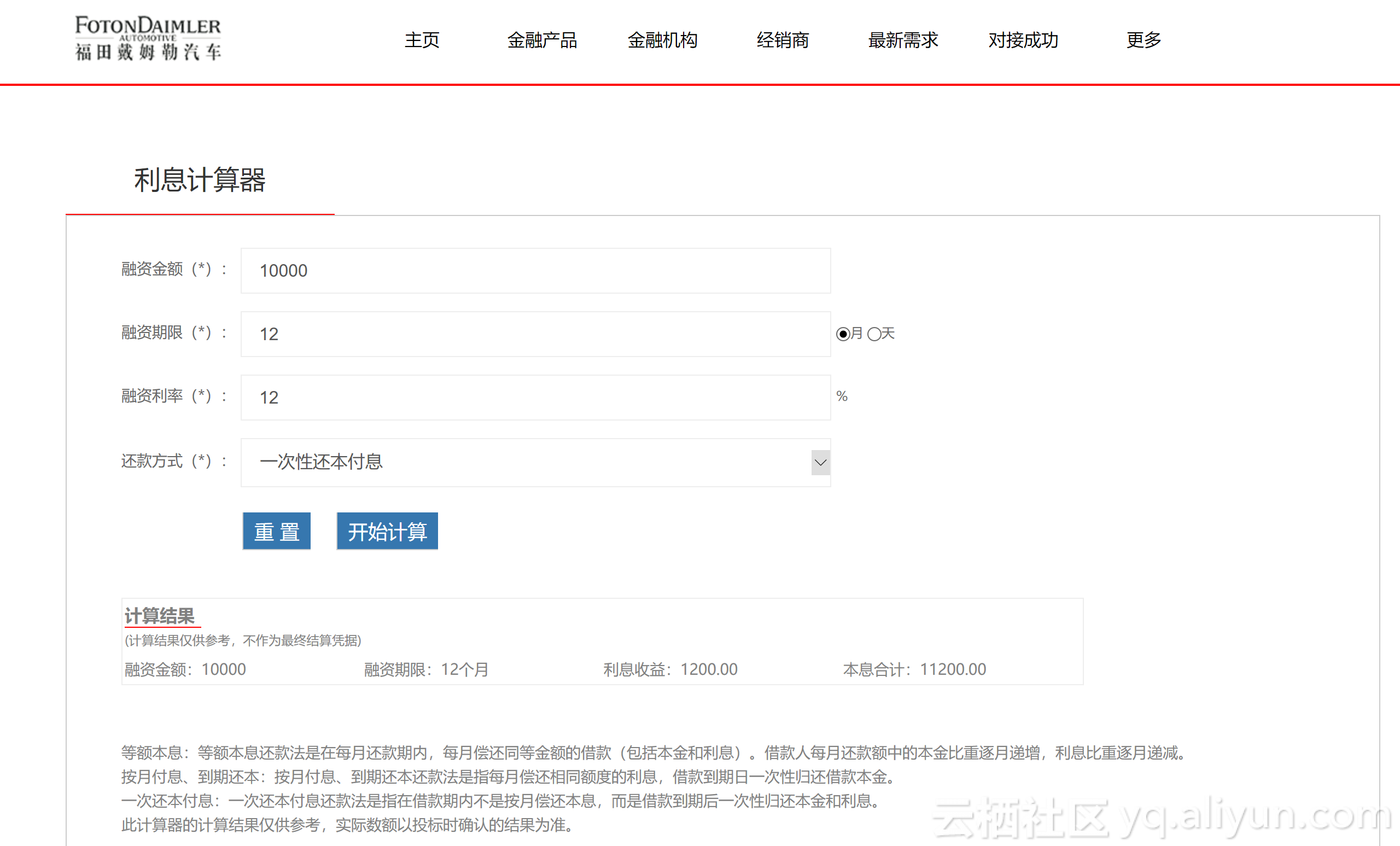import java.util.Scanner;
import java.util.Scanner;
public class InterestComputor{
public static void main(String[] args){
Scanner scanner = new Scanner(System.in);
System.out.println("请输入你的贷款金额");
Double amount = scanner.nextDouble();
System.out.println("请输入你的贷款年利率");
Double rate = scanner.nextDouble();
System.out.println("请输入你的贷款月数");
int months = scanner.nextInt();
//amount*(rate/100.0)*(months/12.0)利息计算公式
Double rateIncome =amount*(rate/100.0)*(months/12.0);
Double totalIncome = amount + rateIncome;
System.out.println("贷款 金额"+amount);
System.out.println("贷款 月数"+months);
System.out.println("贷款年利率"+rate );
System.out.println("需偿还利息"+rateIncome );
System.out.println("本息 合计"+totalIncome );
}
}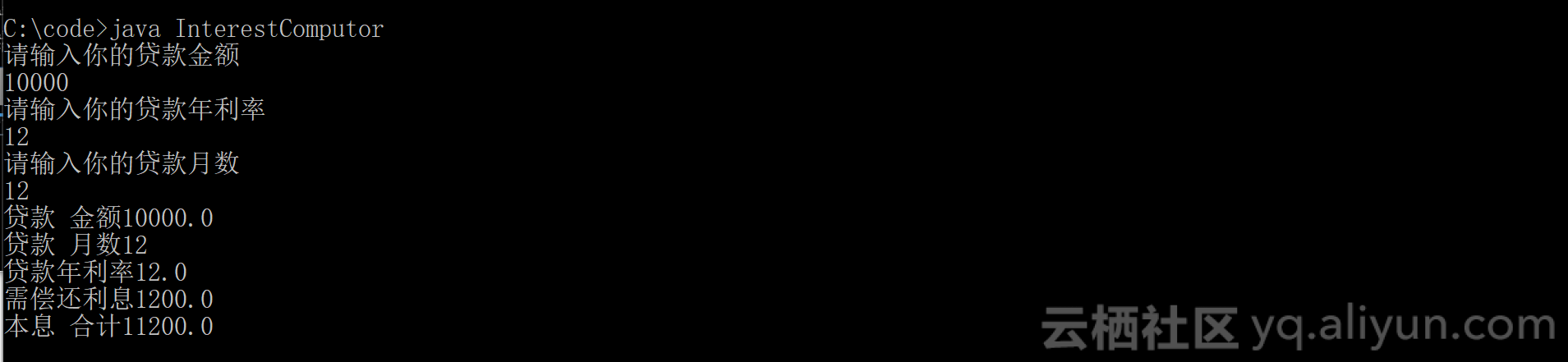// 一次性还本付息

import java.util.Scanner;
public class InterestComputor{
public static void main(String[] args){
Scanner scanner = new Scanner(System.in);
System.out.println("请输入你的贷款金额");
Double amount = scanner.nextDouble();
System.out.println("请输入你的贷款年利率");
Double rate = scanner.nextDouble();
System.out.println("请输入你的贷款月数");
int months = scanner.nextInt();
compute(amount,rate,months);
}
public static void compute(double amount, double rate, int months){
//amount*(rate/100.0)*(months/12.0)利息计算公式
Double rateIncome =amount*(rate/100.0)*(months/12.0);
Double totalIncome = amount + rateIncome;
System.out.println("贷款 金额"+amount);
System.out.println("贷款 月数"+months );
System.out.println("贷款年利率"+rate );
System.out.println("需偿还利息"+rateIncome );
System.out.println("本息 合计"+totalIncome );
}
}

·Java必修课第一讲：https://yq.aliyun.com/articles/604581

·Java必修课第二讲：https://yq.aliyun.com/articles/604584

·Java必修课第三讲：https://yq.aliyun.com/articles/603660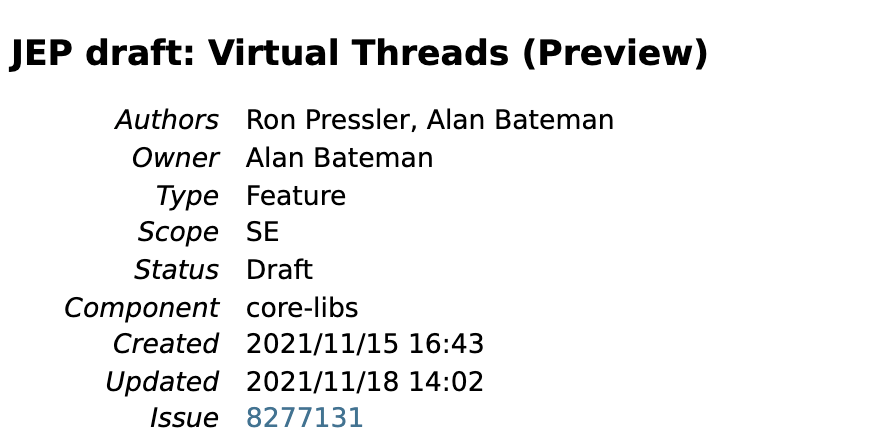98 0Java必读文章 ——《我的Java打怪日记》
java必读！！！
28914 0Java资源大全中文版

3342 0java支付宝开发-00-资源帖

935 0Java正则系列: (2)量词

1062 0Java总结篇系列：Java泛型
722 0Java8初体验系列文章
911 0Java堆内存
Java 中的堆是 JVM 所管理的最大的一块内存空间，主要用于存放各种类的实例对象。   在 Java 中，堆被划分成两个不同的区域：新生代 ( Young )、老年代 ( Old )。
832 0(2)java堆内存
java堆内存结构图 【名词解释】--->eden,so,s1通称为新生代对象储区--->tenured称为老年代对象存储区--->s0和s1也称为from和to区域，是两块大小相等，可以互换角色的内存空间。
823 0
37

0